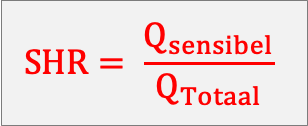BeneluxThe Sensible Heat Ratio (SHR) reflects the ratio of the sensible (perceptible) heat load (Qsensible) and the total heat load (sensible & latent).

Calculation example

A cooling system emits a total amount of heat of 20 KJ/kg to cool the cabin at atmospheric pressure from 35 °C to 26 °C. The air inside the cabin has an average heat of CP of 1.050 KJ/kg.

Qtotal = 13 KJ/kg

Tinitial = 32 °C

Tfinal = 26 °C

CP = 1.050 KJ/kg

The total heat load (Qsensible) can then be calculated:

QSensible = CP (Tinitial – Tfinal)

QSensible = 1.050 (35 – 26)

QSensible = 9.450

Then the SHR is calculated as follows:

SHR = Qsensible / Qtotal

SHR = 9.450 / 13

The SHR amounts to 0.726An SHR lower than 1 means that due to dehumidification of the air, the air conditioning system in a data centre is less efficient than it should be. Typically, dehumidification in a DC is not necessary.